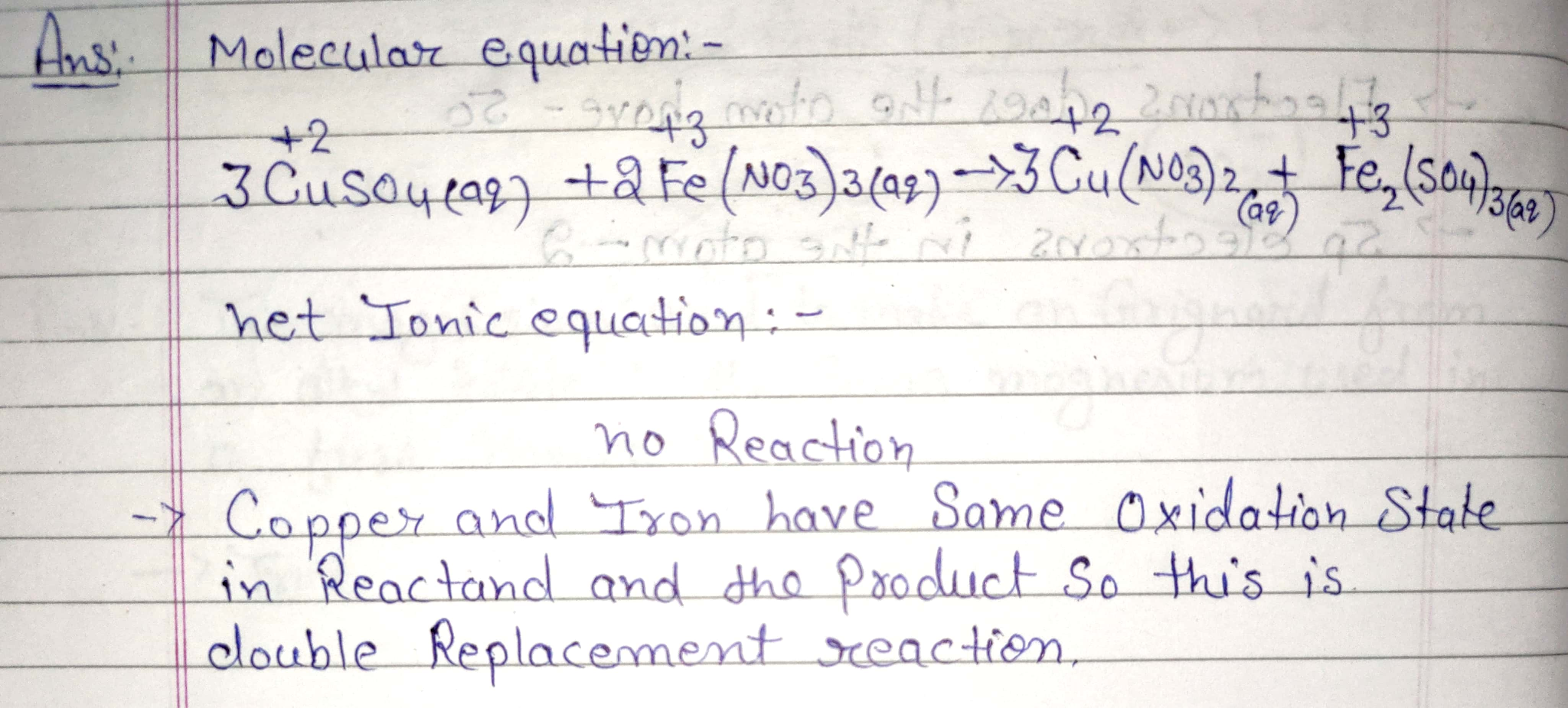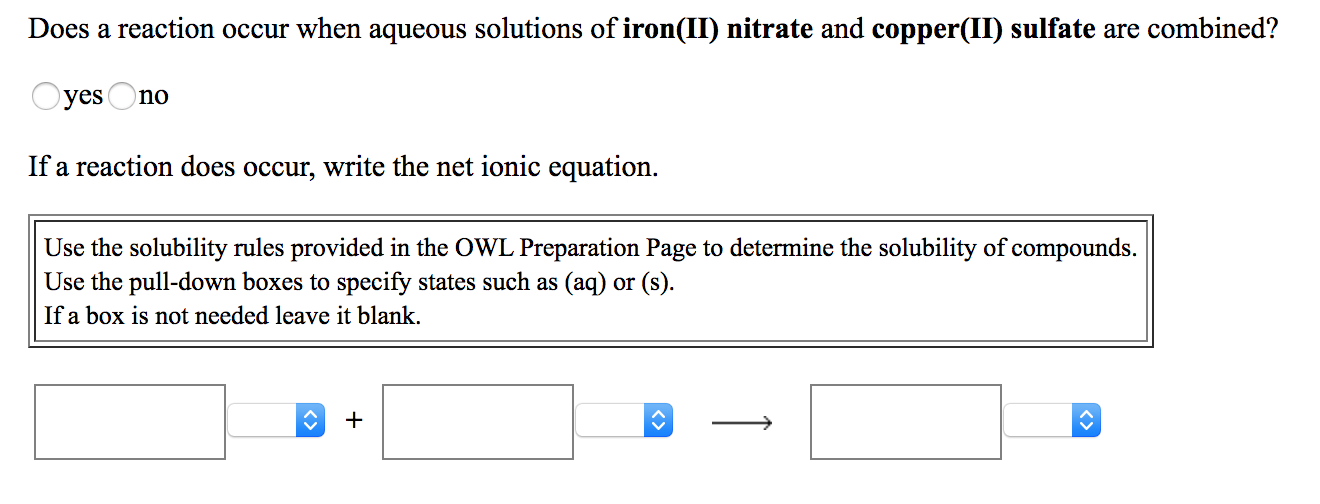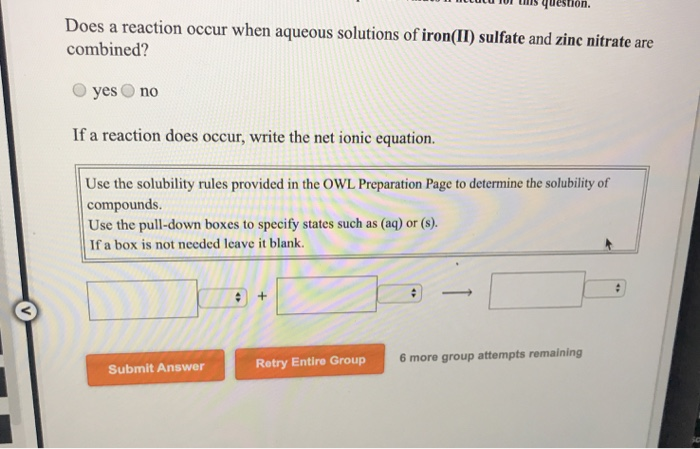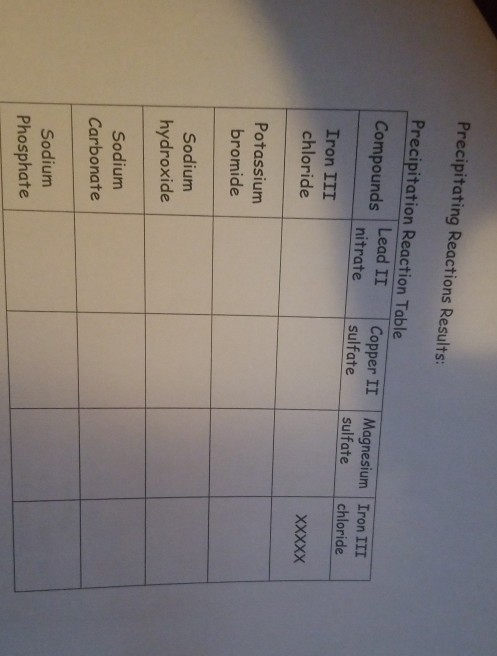# Which best describes the reaction, if any, when aqueous solutions of copper(II) sulfate and iron(III) nitrate...

Which best describes the reaction, if any, when aqueous solutions of copper(II) sulfate and iron(III) nitrate are combined? Play media comment. Group of answer choices copper(II) nitrate and iron(III) sulfate are formed there is no reaction copper is oxidized iron is oxidized#### Earn Coin

Coins can be redeemed for fabulous gifts.

Similar Homework Help Questions
• ### Does a reaction occur when aqueous solutions of iron(II) sulfate and lead(II) nitrate are combined? if...

Does a reaction occur when aqueous solutions of iron(II) sulfate and lead(II) nitrate are combined? if so what is the net ionic reaction? Does a reaction occur when aqueous solutions of manganese(II) nitrate and ammonium sulfate are combined? if so what is the net ionic reaction? Does a reaction occur when aqueous solutions of lead(II) nitrate and copper(II) sulfate are combined?if so what is the net ionic reaction?

• ### Does a reaction occur when aqueous solutions of iron(II) nitrate and copper(II) sulfate are combined? O...Does a reaction occur when aqueous solutions of iron(II) nitrate and copper(II) sulfate are combined? O yes no If a reaction does occur, write the net ionic equation. Use the solubility rules provided in the OWL Preparation Page to determine the solubility of compounds. Use the pull-down boxes to specify states such as (aq) or (s). If a box is not needed leave it blank. Does a reaction occur when aqueous solutions of silver(I) nitrate and manganese(II) sulfate are combined?...

• ### 4 Which best describes the reaction, if any, that occurs when aqueous solutions of silver nitrate...

4 Which best describes the reaction, if any, that occurs when aqueous solutions of silver nitrate and sodium iodide are combined?    AgI3 is formed as a precipitate NaNO3 is formed as a precipitate AgI is formed as a precipitate silver is reduced

• ### 15. Does a reaction occur when aqueous solutions of cobalt(II) chloride and iron(III) nitrate are combined?...

15. Does a reaction occur when aqueous solutions of cobalt(II) chloride and iron(III) nitrate are combined? yesno If a reaction does occur, write the net ionic equation. 16. Does a reaction occur when aqueous solutions of potassium phosphate and chromium(III) chloride are combined? yesno If a reaction does occur, write the net ionic equation. 17. Does a reaction occur when aqueous solutions of silver(I) nitrate and barium chloride are combined? yesno If a reaction does occur, write the net ionic...

• ### Does a reaction occur when aqueous solutions of sodium hydroxide and iron(II) sulfate are combined? if...

Does a reaction occur when aqueous solutions of sodium hydroxide and iron(II) sulfate are combined? if so what is the net ionic equation? Does a reaction occur when aqueous solutions of potassium nitrate and sodium hydroxide are combined? if so what is the net ionic equation? Does a reaction occur when aqueous solutions of chromium(III) nitrate and sodium hydroxide are combined? if so what is the net ionic equation?

• ### 15. Does a reaction occur when aqueous solutions of cobalt(II) chloride and iron(III) nitrate are combined?...

15. Does a reaction occur when aqueous solutions of cobalt(II) chloride and iron(III) nitrate are combined? yesno If a reaction does occur, write the net ionic equation. 16. Does a reaction occur when aqueous solutions of potassium phosphate and chromium(III) chloride are combined? yesno If a reaction does occur, write the net ionic equation. 17. Does a reaction occur when aqueous solutions of silver(I) nitrate and barium chloride are combined? yesno If a reaction does occur, write the net ionic...

• ### 10 luCU TUI is question. Does a reaction occur when aqueous solutions of iron(II) sulfate and...10 luCU TUI is question. Does a reaction occur when aqueous solutions of iron(II) sulfate and zinc nitrate are combined? yes no If a reaction does occur, write the net ionic equation. Use the solubility rules provided in the OWL Preparation Page to determine the solubility of compounds. Use the pull-down boxes to specify states such as (aq) or (8) If a box is not needed leave it blank. 6 more group attempts remaining Submit Answer Retry Entire Group

• ### Consider the reaction when aqueous solutions of iron(II) chloride and lead(II) nitrate are combined. The net...

Consider the reaction when aqueous solutions of iron(II) chloride and lead(II) nitrate are combined. The net ionic equation for this reaction is:

• ### Precipitating Reactions Results: Precipitation Reaction Table Compounds Lead Lead II Copper II nitrate sulfate Iron III...Precipitating Reactions Results: Precipitation Reaction Table Compounds Lead Lead II Copper II nitrate sulfate Iron III chloride Magnesium sulfate Iron III chloride Potassium bromide Sodium hydroxide Sodium Carbonate Sodium Phosphate

• ### Which ion(s) would NOT be spectator ions when aqueous solutions of lead(II) nitrate and potassium iodide...

Which ion(s) would NOT be spectator ions when aqueous solutions of lead(II) nitrate and potassium iodide are combined? Select all that apply. Group of answer choices Pb2+ NO3- K+ I- there is no reaction

Free Homework App